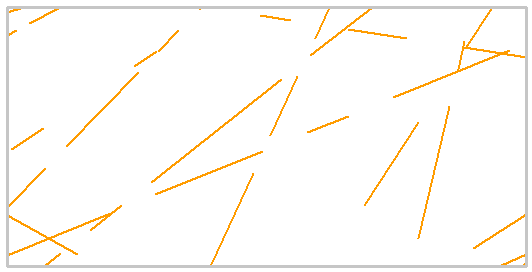RSWebinar: Designing Cost-Effective Ground Improvement Solutions with Settle3 | June 7th, 2023 Register Now

# Joint Model: Veneziano

In a two-dimensional trace plane, the Veneziano joint network model is based on a Poisson line process. It however adapts the Poisson process to generate joints of finite length (Dershowitz, 1985). The process for creating a Veneziano joint network consists of:

1. Generating infinite joint lines, each of which passes through a point located according to a Poisson point process (i.e. points distributed in the trace plane according to a uniform distribution). The orientations of the lines may be constant or vary according to some orientation distribution.
2. Dividing each joint line into segments of random lengths. These lengths correspond to a specified statistical distribution. (In the original Veneziano formulation, lengths were assumed to have an exponential distribution. RS2 relaxes this condition and allows users to specify other statistical distributions.)

A portion of these segments are classified as joints and the remainder as intact rock bridges. The proportion of joints to intact rock bridges is determined by the length persistence parameter.

As a result of the use of joint lines as the first step in generating joints, joints in a Veneziano network tend to be coplanar. (Coplanar joints are joints that are all located in the same plane.) This tendency towards coplanarity differentiates the Veneziano model from the Baecher model in which joints tend to be independent segments.

Veneziano joint network, random joint length, orientation and persistenceThe following input parameters can be defined for the Veneziano joint network model.

## Orientation Definition

The options for defining the joint orientation for the Veneziano joint network model, are exactly the same as the Baecher model. For details see the Baecher joint network topic.

## Joint Length

The Joint Length defines the distribution of joint lengths as measured in the trace plane.

To define the Joint Length as a random variable, choose a Statistical Distribution (Normal, Uniform, Exponential or Lognormal) and enter the distribution parameters.

## Length Persistence

Coplanar joints are separated from each other by intact rock material (rock bridges). In a two-dimensional trace plane, the ratio of joint length to the sum of joint length and rock bridge length is known as Length Persistence. The Persistence is a measure of joint continuity and can vary between 0 and 1.

To define the Length Persistence as a random variable, choose a Statistical Distribution (Normal, Uniform, Exponential or Lognormal) and enter the distribution parameters.

## Joint Intensity

Joint intensity measures describe the degree of jointing that occurs within a volume of rock mass. There are five measures for joint intensity in two dimensions. These are:

1. P1 = the number of joint traces per unit area of the trace plane
2. P2 = the sum of joint trace lengths per unit area of the trace plane
3. P3 = the sum of joint trace lengths per square root of the trace plane area
4. P4 = the sum of squared joint trace lengths per unit area
5. P5 = the square of the sum of joint lengths per unit area

The last three measures are dimensionless.

## Joint End Condition

For a description of the Joint End Condition option, see the Add Joint Network topic. For further information see the Add Joint Boundary topic.

## Randomize

If you select the Randomize button, the Veneziano joint network will be re-generated, using a new sampling of the random variables (e.g. joint orientation, joint length, joint persistence).